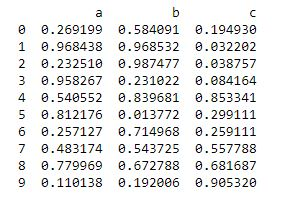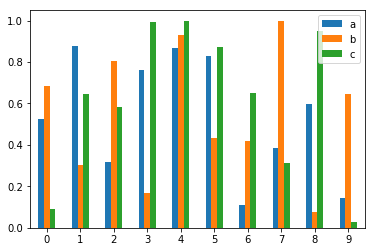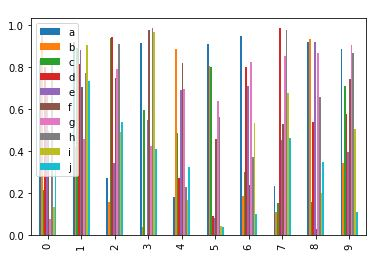# Python | Pandas Dataframe.plot.bar

Python is a great language for doing data analysis, primarily because of the fantastic ecosystem of data-centric python packages. Pandas is one of those packages and makes importing and analyzing data much easier.

Pandas `DataFrame.plot.bar()` plots the graph vertically in form of rectangular bars.

Syntax : DataFrame.plot.bar(x=None, y=None, **kwds)

Parameters:
x : (label or position, optional) Allows plotting of one column versus another. If not specified, the index of the DataFrame is used.
y : (label or position, optional) Allows plotting of one column versus another. If not specified, all numerical columns are used.

Returns: matplotlib.axes.Axes or np.ndarray of them

Example #1: Using `DataFrame.plot.bar()` to plot the graph vertically in form of rectangular bars

 `# importing matplotlib ` `import` `matplotlib.pyplot ` ` `  `# importing pandas as pd ` `import` `pandas as pd ` ` `  `# importing numpy as np ` `import` `numpy as np ` ` `  `# creating a dataframe  ` `df ``=` `pd.DataFrame(np.random.rand(``10``, ``3``), columns ``=``[``'a'``, ``'b'``, ``'c'``]) ` ` `  `print``(df) `Now we will use a function `DataFrame.plot.bar()` to plot a graph vertically in form of rectangular bars

 `# using a function df.plot.bar() ` `df.plot.bar() `

Output:Example #2: Using `DataFrame.plot.bar()` to plot the graph vertically in form of rectangular bars.

 `# importing matplotlib ` `import` `matplotlib.pyplot ` ` `  `# importing pandas as pd ` `import` `pandas as pd ` ` `  `# importing numpy as np ` `import` `numpy as np ` ` `  `# creating a dataframe ` `df ``=` `pd.DataFrame(np.random.rand(``10``, ``10``), ` `                  ``columns ``=``[``'a'``, ``'b'``, ``'c'``, ``'d'``, ``'e'``, ``'f'``, ``'g'``, ``'h'``, ``'i'``, ``'j'``]) ` ` `  `df `Now we will use a function `DataFrame.plot.bar()` to plot a graph vertically in form of rectangular bars

 `# using a function df.plot.bar() ` `df.plot.bar() `

Output :My Personal Notes arrow_drop_upCheck out this Author's contributed articles.

If you like GeeksforGeeks and would like to contribute, you can also write an article using contribute.geeksforgeeks.org or mail your article to contribute@geeksforgeeks.org. See your article appearing on the GeeksforGeeks main page and help other Geeks.

Please Improve this article if you find anything incorrect by clicking on the "Improve Article" button below.

Article Tags :

Be the First to upvote.

Please write to us at contribute@geeksforgeeks.org to report any issue with the above content.